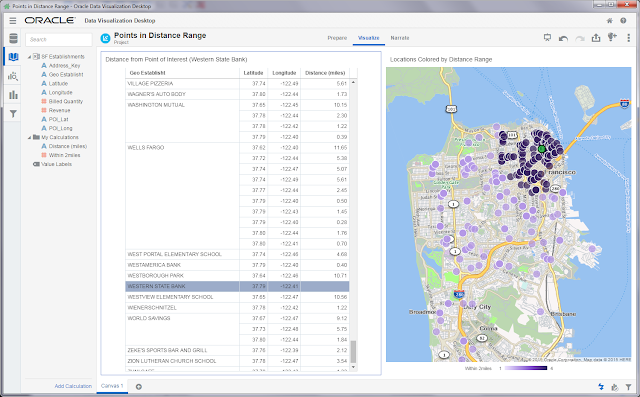Monday, February 20, 2017

OracleDV : Calculating distance using latitude/Longitude

In this blog we will talk about how to compute distance between two points using latitude and longitude using inbuilt functions in Oracle DV. In Geospatial Analysis, requirement to compute distance between two points using latitude and longitude is quite prevalent. Haversine formula is frequently used to calculate distance between two points on earth using latitudes and longitudes. Haversine formula computes great circle distance(distance as measured along the surface of earth/sphere rather than the distance through the sphere/earth). This formula is based on a generic formula in Spherical trignometry, called law of haversines. Following is the formula:

Following is the calculation in OracleDV to compute the distance between two lat longs using Haversine formula:

CASE
WHEN Source_Lat=Dest_Lat AND Source_Long=Dest_Long
THEN 0
ELSE
ACOS(
6371
END

In this formula:
Source_lat refers to Source Latitude
Source_Long refers to Source Longitude
Dest_Lat refers to Destination Latitude
Dest_Long refers to Destination Longitude

Please note that Source and destination are used only for naming convenience, they can actually be used interchangeably. Distance computed using lat long may differ from the actual driving distance between two points depending on various factors such as road connectivity and presence of other geographic bodies. Here is a snapshot of the project on Oracle DV Desktop.